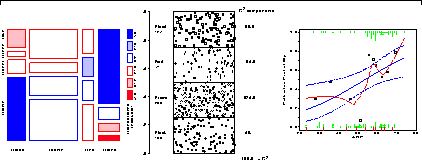# Chapter 1. IntroductionCategorical data consists of variables whose values comprise a set of discrete categories. Such data require different statistical and graphical methods than commonly used for quantitative data. The focus of this book is on visualization techniques and graphical methods designed to reveal patterns of relationships among categorical variables. 1.1. Data visualization and categorical data 1.2. What is categorical data? 1.2.1. Case form vs. Frequency form 1.2.2. Frequency data vs. Count data 1.2.3. Univariate, bivariate, and multivariate data 1.2.4. Explanatory vs. Response variables 1.3. Strategies for categorical data analysis 1.3.1. Hypothesis testing approaches 1.3.2. Model building approaches 1.4. Graphical methods for categorical data 1.4.1. Goals and design principles for visual data display 1.4.2. Categorical data require different graphical methods 1.5. Visualization = Graphing + Fitting + Graphing 1.5.1. Static vs. dynamic graphics